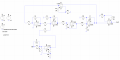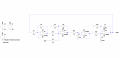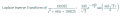#### richard_lai

Joined Nov 21, 2019
24
Hi,

In that circuit first get rid of that input stage added to Vin.
Then change C6 and C7.

I looked at the theory with the two extra gain stages and the steady state error goes down lower as the gains go up.

But in your circuit there i think you can change C6 and C7 by a factor of 1/10 and get better results, but you can try lower too. So instead of 2.2uf use 0.22uf and see if you like the results both in theory and in the simulation. If not you may have to go lower.
Going down in C by a factor of 1/10 means the steady state error goes down by a factor of 1/10^2 which is 1/100. So if you stated with a SS error of 0.366 it will go down to 0.00366.

The key point is increasing the gain lowers the steady state error.
So the gain of the op and the output side will be the same?

#### MrAl

Joined Jun 17, 2014
8,556
So the gain of the op and the output side will be the same?
Yes. Make them the same, start with maybe a gain of 2 and go up and see how much difference it makes in the SS error.

#### richard_lai

Joined Nov 21, 2019
24
Hi,

In that circuit first get rid of that input stage added to Vin.
Then change C6 and C7.

I looked at the theory with the two extra gain stages and the steady state error goes down lower as the gains go up.

But in your circuit there i think you can change C6 and C7 by a factor of 1/10 and get better results, but you can try lower too. So instead of 2.2uf use 0.22uf and see if you like the results both in theory and in the simulation. If not you may have to go lower.
Going down in C by a factor of 1/10 means the steady state error goes down by a factor of 1/10^2 which is 1/100. So if you stated with a SS error of 0.366 it will go down to 0.00366.

The key point is increasing the gain lowers the steady state error.

[LATER]
The cap change idea may only work for the previous circuit not this latest one. Try the two gains first so you can at least see it work like that.
I had tried both and all works. Now the circuit before the summing amplifier dosent need anymore to eliminate the error. Just change the value of capacitor or add a gain at the feedback path and the output. When the gain is more than 2, it become more worst when the gain increase. The best for it is 2.

When i add a gain at the feedback path, why i also need to add at the output? Dosent it amplify the signal more?

Can I know the theory behind both of the method which one is change the capacitor value and another one is the gain? Any article about it?

#### MrAl

Joined Jun 17, 2014
8,556
I had tried both and all works. Now the circuit before the summing amplifier dosent need anymore to eliminate the error. Just change the value of capacitor or add a gain at the feedback path and the output. When the gain is more than 2, it become more worst when the gain increase. The best for it is 2.

When i add a gain at the feedback path, why i also need to add at the output? Dosent it amplify the signal more?

Can I know the theory behind both of the method which one is change the capacitor value and another one is the gain? Any article about it?
Hi,

You must be doing something wrong so you are going to have to show your most recent circuit or else i will show mine. In theory the two gains going higher decrease the steady state error and you can see the simulations i did clearly shows that works.

When we increase the gain in the feedback path we effectively reduce the total output amplitude so we need another gain stage at the very output to get the original overall gain back to normal.

The theory in this case involves looking at the transfer function and seeing which gain or gains will decrease the steady state error. We can look at this more too.

Here is the system i used to simulate the result after the two new gains were added. Ignore V1.

Oh BTW i had to use the Inverse Laplace Transform to calculate the steady state error. When i tried to use the s*E method it did not produce any useful results. I have not investigated why this does not work with one of the systems that shows lower SS error with increase in one or more gains.

Last edited:

#### richard_lai

Joined Nov 21, 2019
24
Hi,

You must be doing something wrong so you are going to have to show your most recent circuit or else i will show mine. In theory the two gains going higher decrease the steady state error and you can see the simulations i did clearly shows that works.

When we increase the gain in the feedback path we effectively reduce the total output amplitude so we need another gain stage at the very output to get the original overall gain back to normal.

The theory in this case involves looking at the transfer function and seeing which gain or gains will decrease the steady state error. We can look at this more too.

Here is the system i used to simulate the result after the two new gains were added. Ignore V1.

Oh BTW i had to use the Inverse Laplace Transform to calculate the steady state error. When i tried to use the s*E method it did not produce any useful results. I have not investigated why this does not work with one of the systems that shows lower SS error with increase in one or more gains.
For my current circuit, the circuit with gain will be like this:And for the one with 0.22u capacitor is thisBoth works for me now and the gain i use which best fit my graph is with a gain of 2.

When changing the gain more bigger for my circuit the output starts to lag the input.

So you mean when we add a gain at the feedback path, we need to add output to return the output back to original? Doesnt that two gain should be added together? So it is different for this case right?

What about the capacitor one? whats the theory behind the method?

Can you show me how u calculate the error by inverse Laplace transform?

#### MrAl

Joined Jun 17, 2014
8,556
Hi,

Your circuits look ok but you need to get rid of that extra stage that is connected to Vin that is not good. That may be why you cant go above a gain of 2 with that circuit. That stage messes things up.

The error by ILT is done simply by assuming a ramp input with amplitude 5 and time 0.030 seconds, then taking the Inverse Laplace Transform, then separating that into three terms, then looking at the three terms and noting that for t=0.030 and gain=1 one terms is near zero already and one term is around 0.166 volts and one term is 5v. The 0.166 term is the steady state error term. Now increasing the gain (or letting the value of the last integrator C go down by a factor C/g where g is the gain) to 10, the 0.166 term goes down significantly which means the steady state error went down. Doing a simulation confirms this as the two waves input and output start to look nearly the same.

If you want to see this happen though with both circuits you are going to have to get rid of that input stage you added before the one that connects to Vin and acts more or less like a differentiator. Get rid of that or else it will not work as well as it should.

#### richard_lai

Joined Nov 21, 2019
24
Hi,

Your circuits look ok but you need to get rid of that extra stage that is connected to Vin that is not good. That may be why you cant go above a gain of 2 with that circuit. That stage messes things up.

The error by ILT is done simply by assuming a ramp input with amplitude 5 and time 0.030 seconds, then taking the Inverse Laplace Transform, then separating that into three terms, then looking at the three terms and noting that for t=0.030 and gain=1 one terms is near zero already and one term is around 0.166 volts and one term is 5v. The 0.166 term is the steady state error term. Now increasing the gain (or letting the value of the last integrator C go down by a factor C/g where g is the gain) to 10, the 0.166 term goes down significantly which means the steady state error went down. Doing a simulation confirms this as the two waves input and output start to look nearly the same.

If you want to see this happen though with both circuits you are going to have to get rid of that input stage you added before the one that connects to Vin and acts more or less like a differentiator. Get rid of that or else it will not work as well as it should.
So you mean the three term to inverse is from the equation earliest right? one is 198025/s^2,another one is 198025/445s and the last one is 198025/198025? Then where does the amplitude need to be substituted?

As when i use online calculator for the equation, it gives me very weird equation.#### MrAl

Joined Jun 17, 2014
8,556
So you mean the three term to inverse is from the equation earliest right? one is 198025/s^2,another one is 198025/445s and the last one is 198025/198025? Then where does the amplitude need to be substituted?

As when i use online calculator for the equation, it gives me very weird equation.
View attachment 193272
[LATER]
I got an expression for the steady state error for the system without the two gains just changing the gain of the last stage by changing either one cap (in the single integrator form) or both caps (in the double integrator form you had been using since day 1):
SSE=(a*C*R)/T
From this we immediately see that as we reduce C or R or both the SSE goes down.
(a and T are constants related to the input ramp signal).
Note C only changes in the very last stage alone.

Hi,

You are definitely on the right track. You should have however incorporated the Vin signal with your transfer function unless of course you have it in the first form shown below. I am not sure what you did there so i will show this in a more general way using constants.

The transfer function you would have come up with would have the form like this:
Vout/Vin=A/(s^2+B*s+C)
where A, B, C are general constants.

You then just multiply by Vin and get:
Vout=A*Vin/(s^2+B*s+C)

You then just substitute:
Vin=a/(T*s^2)

where:
a is the amplitude of the ramp at the top (5v in this problem), and
T is the first time that the input reaches that amplitude 'a' (0.030 seconds in this problem).

Doing that we end up with:
Vout=(a/(T*s^2))*A/(s^2+B*s+C)

which of course comes out to:
Vout=(a*A)/(s^2*(C+s*B+s^2)*T)

Now you can go with that or substitute a=5 and T=0.030 and the other constants as they appear in the actual analysis. Then take the Inverse Laplace Transform.
You get three terms if you simplify, and see if you can spot the steady state error term.
Setting t=0.030 or possibly t=0.015 would give you the numerical value (see below).

I did that and let the gain 'g' be a variable, then at the end i plotted the steady state error and you can see it go down down down as the gain gets larger.

There is also a more intuitive way to approach this but you have to be careful to select the evaluation time right. This involves subtracting the time expressions Vin and Vout as:
Vin(t)-Vout(t)

and then setting the evaluation time to somewhere between the peaks. In this problem that might be 0.015 seconds but again you have to be careful to check the graphs of the two functions to make sure that's a reasonable place to do the evaluation. The subtraction yields the approximate steady state error. If you do a simulation and the time to do the evaluation looks like it is several cycles from t=0 though, you'll have to go through a lot more trouble to include initial values in the analysis and do several half cycles one after the other until you reach the point in time where you want to d the evaluation. That can be quite involved but it is more intuitive to think of it that way.

Last edited:

#### MrAl

Joined Jun 17, 2014
8,556
A couple more little notes...

The general procedure would be to first check to see if the order of the system matches or exceeds the order (or degree) of the input signal. In order for the system to track a polynomial of degree 2 the system has to be at least of order 2. If the degree of the input poly matches that of the system order, then the steady state error can be made small but not zero. If the order of the system exceeds the degree of the input poly then the steady state error can be reduced to zero.
Sometimes we can get very close to zero though even if the degree of the input is the same as the order of the system. That's what we have been doing here.

If the system is not of sufficient order, then we add an integrator and proportional constant in the path just after the error signal. The error signal is the place in the circuit where we calculate (using the op amp) Vin minus Vout.
With this idea the question comes up as to where we might put the gain when the system order is equal to the input degree. It would appear that it could be anywhere after the error signal, but i have not investigated that. It does make sense though because by increasing the integrator gain we effectively added a constant gain in the path just after the error signal. That's why the SS error went down. If the system order was less than the degree of the input however, that would not work as we would also have to add an integrator along with the constant gain, and the integrator would raise the order of the system by 1.

The order of the system is usually the number of cascaded integrators. However, since one of your circuits uses a pole zero cancelation technique the theoretical system is only of order 2 even though there appears to be 3 integrators. This should change also though, to a system with only 2 integrators and only 2 integrators visible by casual observation. Pole zero cancelation techniques should be avoided.

So with all that said, we see a general procedure emerge...
Check to see that the degree of the input polynomial is greater than the order of the system.
If it is, one or more integrators have to be added just after the error signal in that signal path.
If the degree is higher by 1, then just add one integrator, if it is higher by 2, add two integrators, if it is higher by N then add N integrators. This ensures a low non zero SS error.
To get a zero SS error, add N+1 integrators.
Along with the integrators add a constant gain.

So if we call the degree of the input D and the order O, we do the following:

If we want low SS non zero error then:
If D=O just change the gain.
If D>O add D-O integrators, add a constant gain also or just change the existing gain.

If we want zero SS error then:
If D>=O add D-O+1 integrators, and add a constant gain or change the existing gain.

For all of these cases we are talking about the signal path just after the error signal (Vin-Vout).
Since that may be a single path, we could probably add or change things anywhere in that path with attention to the practical circuit power supply constraints.

Last edited: